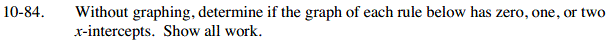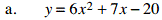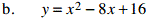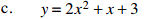### Home > AC > Chapter 10 > Lesson 10.2.4 > Problem10-84A delete

10-84A delete.The graphs of these equations are all parabolas. To find the x-intercepts, let y = 0 and solve using the Quadratic Formula or factoring and the Zero Product Property.0 = (3x − 5)(x + 4)

Two intercepts.0 = (x − 4)2Zero intercepts.